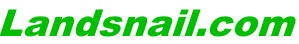Apple II Programmer's Reference

][ in a Mac Emulator

Old Computer Book Gallery

Ray Borrill & The Data Domain

Apple II Programmer's Reference

Here's a brief list of Applesoft, Integer Basic, DOS 3.3, and ProDOS commands with descriptions.

I found these in the back of a manual for II in a Mac, one of the first Apple II emulators that ran on the original Mac and Mac 512. It came out in 1985.

 APPLESOFT Reference Statements and Lines A line typed without a line number is executed immediately; those lines typed with a line number are saved for execution with the RUN command. : Separates multiple statements on the same line. REM Designates a remark for comments. Operations on Whole Programs NEW Erases the current program and clears all variables. CLEAR Resets all variables. LIST Displays the entire current program. LIST 11-12 Displays the lines from 11 to 12. RUN Executes the current program. RUN n Executes the current program from line n. LOAD Loads a program from the cassette I/O port. SAVE Saves a program to the cassette I/O port. Variables Real AB +/- 9.9999999 E+37 Integer AB% +/- 32767 String AB\$ 0 to 255 characters Arrays Real AB(x,y,z) Integer AB%(x,y,z) String AB\$(x,y,z) Where A is a letter, B is a letter or digit. The size of an array is limited only by the available memory. DIM a (x,y,z) Defines the array a with maximum subscripts of x, y and z. Arithmetic Operators = Assigns a value to a variable - Subtraction + Addition / Division * Multiplication ^ Exponentiation Logical Operators AND Both true OR Either one or both true NOT Is false Relational Operators = Equal to < Less than > Greater than <= =< Less than or equal to >= => Greater than or equal to <> >< Not equal to Arithmetic Functions ABS(x) Absolute value of x SGN(x) -1 if x<0, 0 if x=0, 1 if x>0 INT(x) Integer portion of x SQR(x) Square root of x SIN(x) Sine of x COS(x) Cosine of x TAN(x) Tangent of x ATN(x) Arctangent of x EXP(x) e raised to the xth power LOG(x) Natural logarithm of x RND(x) if x>0, random number between 0 and 1 if x=0, repeats last random number if x<0, begins new repeatable sequence DEF FN (x) = expr Defines a function String Operations + Concatenates Strings LEN(s) Length of string s LEFT\$(s,x) Leftmost x characters of string s MID\$(s,x,y) y characters from s, beginning at position x RIGHT\$(s,x) Rightmost x characters of string s STR\$(x) String representing x VAL(s) Numeric value of string s CHR\$(x) Character with ASCII code x ASC(s) ASCII code for first character in string s Control GOTO n Branches to line n ON expr GOTO n1,n2,n3... Branches to line n1,n2,n3...depending on the value of expr IF cond THEN s1:s2:s3 Executes statements s1,s2,s3...if expr is true IF expr GOTO line Shorthand for "IF expr THEN GOTO line", but the interpreter remembers the syntax (it doesn't tokenize it as an if-then-goto, but rather as if-goto) FOR v=x TO y STEP z Begins a loop for all values of v from x to y by z; if step is omitted, 1 is understood. NEXT v Repeats loop for next value of v GOSUB n Branches to subroutine at line n RETURN Returns to point of call from a subroutine ON expr GOSUB n1,n2,n3... Branches to subroutine at line n1,n2,n3...depending on the value of expr POP Removes one address from the return stack ONERR GOTO n Sets the line number branched to when an error occurs. RESUME Re-executes statement causing an error. STOP Halts program and print line number CONT Resumes program execution END Halts program execution Utility Statements PEEK(addr) Value of memory location addr POKE addr,x Sets memory location addr to x CALL addr Executes machine language routine at addr USR(x) Passes argument to machine language routine HIMEM:addr Sets highest available memory to addr LOMEM:addr Sets lowest available memory to addr. FRE(0) Amount of available storage TRACE Displays the number of each executed line NOTRACE Turns line numbering off Graphics GR Sets lores graphics mode and clears screen COLOR=x Sets lores drawing color to x PLOT x,y Draws a dot at location x,y HLIN x1,x2 at y Draws a horizontal line from x1,y to x2,y VLIN y1,y2 at x Draws a vertical line from x,y1 to x,y2 SCRN(x,y) Color on the screen at x,y HGR Displays hires page1, mixed mode. HGR2 Displays hires page2, full screen graphics HCOLOR=x Sets hires drawing color to x HPLOT x,y Plots a dot at coordinate x,y HPLOT x1,y1 TO x2,y2 Draws a hires line from x1,y1 to x2,y2 DRAW n AT x,y Draws shape n at coordinate x,y XDRAW n AT x,y Draws shape n at x,y using exclusive-or SCALE=x Sets scale to x for shape drawing ROT=x Sets rotation to x for shape drawing Input/Output IN# n Re-directs input from slot number n INPUT s;x,y,... Prompts with string s, then reads x,y,... GET c Reads a single character from keyboard READ x,y,... Reads values from DATA list into x,y,... DATA x,y,... List of data value RESTORE Restarts DATA list from beginning PDL(n) Value of paddle n, either 0 or 1 PR# n Re-directs output to slot number n PRINT x,y,... Prints values x,y,... ? Shorthand for PRINT TEXT Sets text mode HOME Clears the text screen HTAB x Sets cursor horizontal to x VTAB x Sets cursor vertical to x INVERSE Sets text printing to black on white NORMAL Sets text printing to white on black FLASH Makes text flash on screen Links to other command sets: Integer Basic | DOS 3.3 | ProDOS
July 7, 2009